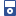Accessibility options:

# Simultaneous linear equations resources

Show me all resources applicable to

### iPOD Video (7)Simultaneous Linear Equations Part 1
IPOD VIDEO: The purpose of this section is to look at the solution of simultaneous linear equations. We will see that solving a pair of simultaneous equations is equivalent to finding the location of the point of intersection of two straight lines. This resource is released under a Creative Commons license Attribution-Non-Commercial-No Derivative Works and the copyright is held by Skillbank Solutions Ltd.Simultaneous Linear Equations Part 2
IPOD VIDEO: The purpose of this section is to look at the solution of simultaneous linear equations. We will see that solving a pair of simultaneous equations is equivalent to finding the location of the point of intersection of two straight lines. This resource is released under a Creative Commons license Attribution-Non-Commercial-No Derivative Works and the copyright is held by Skillbank Solutions Ltd.Simultaneous Linear Equations Part 3
IPOD VIDEO: The purpose of this section is to look at the solution of simultaneous linear equations. We will see that solving a pair of simultaneous equations is equivalent to finding the location of the point of intersection of two straight lines. This resource is released under a Creative Commons license Attribution-Non-Commercial-No Derivative Works and the copyright is held by Skillbank Solutions Ltd.Simultaneous Linear Equations Part 4
IPOD VIDEO: The purpose of this section is to look at the solution of simultaneous linear equations. We will see that solving a pair of simultaneous equations is equivalent to finding the location of the point of intersection of two straight lines. This resource is released under a Creative Commons license Attribution-Non-Commercial-No Derivative Works and the copyright is held by Skillbank Solutions Ltd.Simultaneous Linear Equations Part 5
IPOD VIDEO: The purpose of this section is to look at the solution of simultaneous linear equations. We will see that solving a pair of simultaneous equations is equivalent to finding the location of the point of intersection of two straight lines. This resource is released under a Creative Commons license Attribution-Non-Commercial-No Derivative Works and the copyright is held by Skillbank Solutions Ltd.Simultaneous Linear Equations Part 6
IPOD VIDEO: The purpose of this section is to look at the solution of simultaneous linear equations. We will see that solving a pair of simultaneous equations is equivalent to finding the location of the point of intersection of two straight lines. This resource is released under a Creative Commons license Attribution-Non-Commercial-No Derivative Works and the copyright is held by Skillbank Solutions Ltd.Simultaneous Linear Equations Part 7
IPOD VIDEO: The purpose of this section is to look at the solution of simultaneous linear equations. We will see that solving a pair of simultaneous equations is equivalent to finding the location of the point of intersection of two straight lines. This resource is released under a Creative Commons license Attribution-Non-Commercial-No Derivative Works and the copyright is held by Skillbank Solutions Ltd.

Website design by Pink Mayhem, Leicester